Module 11 - Extreme Values and Optimization

Introduction | Lesson 1 | Lesson 2 | Lesson 3 | Lesson 4 | Self-Test

Lesson 11.3: The Can Problem

In Lesson 11.2 the length of a removed arc that maximized the volume of a cone was found. In this lesson the radius of a can that minimizes surface area will be explored.

• Execute NewProb from the F6:Clean Up menu

Stating the Problem

A right cylindrical can is being designed to hold 255 cubic units. Find the radius of the can with the least surface area and find the minimum surface area.

Drawing the Diagram

The diagram below illustrates a can of radius r and height h.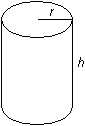Finding Surface Area as a Function of r and h

The quantity to be minimized is surface area. The surface area includes the lateral surface formed by a rectangle with height h and width 2r (the circumference of the circular base) and two circles that form the top and bottom. Therefore, the surface area is the sum of the areas of the two circles and the rectangle: S = 2r2 + 2rh.

• Define s(x)=2**(r(x))^2+2**r(x)*h(x)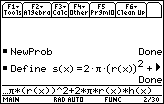Defining r and h as Functions of x

The surface area is a function of two variables, r and h. If r and h are both defined in terms of a single variable x, then the surface area will be defined as a function of x, which will allow it to be graphed on the TI-89.

Writing h and r as Functions of x

The desired volume is 255 cubic units and the formula for volume is V =r2h. Therefore, 255=r2h.

Solving the equation for h gives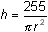Letting r = x, r and h can be defined as functions of x.

• Define r(x)=x
• Define h(x)=255/(*(r(x))^2)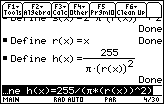Now we have surface area s(x), completely defined as a function of x, as shown in the following screen.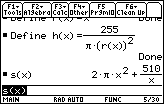Finding the Derivative of Surface Area with respect to x

Store the derivative of the surface area, s, in a new function named ds.

• Define ds(x)=d(s(x),x)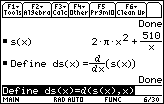Alternatively, we could compute the derivative of s(x) directly from the formula found in the previous figure.

Finding the Zero(s) of the Derivative

Because the radius of the can must be greater than zero, the domain of the surface area function is all x > 0. Notice that if r = k is very large, then h is very small.

Find the exact value and the approximate value of the zero of the derivative.

• Solve(ds(x)=0,x) to get the exact value (there is only one in this case)
• Get the approximate value by pressing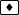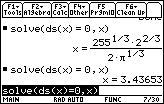Checking for Other Possible Extreme Values

The first derivative is defined everywhere in the function's domain, so x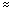3.43653 is the only critical point. There are no endpoints to consider.

Using the Second Derivative

The second derivative can often be used to determine if a critical point where the first derivative is zero indicates a local maximum or a minimum by revealing the function's concavity at that point. No conclusion can be drawn if the second derivative is zero or undefined at the critical point. The table below summarizes the relationship.

 Second Derivative Function Max/Min positive concave upward local minimum negative concave downward local maximum

Test the critical point in the second derivative of the surface area function to determine whether it represents a local minimum. Use the exact value from the history screen.

• d(s(x),x,2)| x =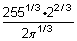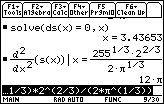Because the second derivative of the surface area function is positive at x = 3.43653, the graph of the function is concave upward and has a local minimum value there.

Because there are no endpoints and no other critical points, the local minimum value is the absolute minimum value.

Finding the Minimum Surface Area

Find the minimum surface area of the can by evaluating s(x) with the exact x-value that minimizes surface area. Then get the approximate value of the minimum surface area.

• Enter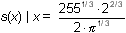The needed x-value is in the History Area, so you can paste it from there to the Edit Line.

• Approximate the last result by pressing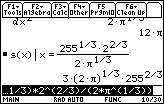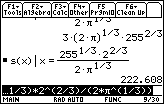Exact Result Approximate Result

The minimum surface area of a cylindrical can that holds 255 cubic units is approximately 222.61 square units. The radius which will result in the minimum surface area is approximately 3.43653 units.

Graphing the Surface Area Function and Its First Derivative

As shown in Lesson 11.2, the graphs of the function and its first derivative illustrate the relationship between the function's minimum value and the corresponding zero of its derivative.

11.3.1

1. Display the graphs of the surface area function and its derivative in a [0, 7] x [-200, 400] window with xscl = 1 and yscl = 50.
2. Use the Zero feature to find the zero of the derivative.
3. Find the minimum value of the surface area graph by using the Minimum feature.
4. Interpret the values found in parts b. and c.
5. Describe the connection between the minimum value of the surface area, the zero of its first derivative, and the value of its second derivative.

Generalizing and Extending the Can Problem

The problem can be generalized by replacing the fixed volume of 255 with a letter and proceeding as in the last example. The problem can be further extended by exploring the ratio of height to radius for a right cylindrical can with fixed volume that has minimum surface area.

Replacing 255 with v

The first occurrence of the fixed value of the volume appears in the function that represents the height of the can. Replace the volume of the can with the letter "v" and redefine the function.

• Copy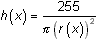from the History Area and paste it in the Edit Line
• Change 255 to v and execute the command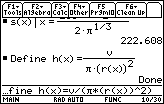Finding The Zero(s) of the Derivative

Determine when the derivative is zero.

• Solve(ds(x)=0,x)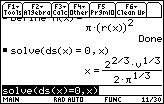This value of x is the radius which will result in the minimum surface area.

• Evaluate s(x) at this value of x.
•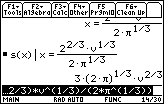The corresponding value of the minimum surface area is s = 3(2)1/3v2/3.

Finding the Ratio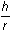Evaluate the ratio of height to radius,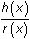, at the zero of the derivative.

• Enter the command h(x)/r(x) | x=2^(2/3)*v^(1/3)/(2*^(1/3))
• Paste the last part of the command from the History Area.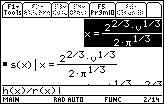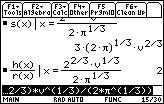The ratio of height to radius is 2, regardless of the volume v. That is, for right cylindrical cans with a fixed volume, when the height of the can is twice the radius the surface area of the can will be minimized.

< Back | Next >